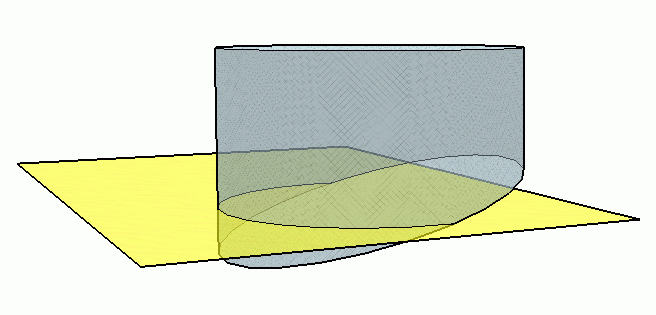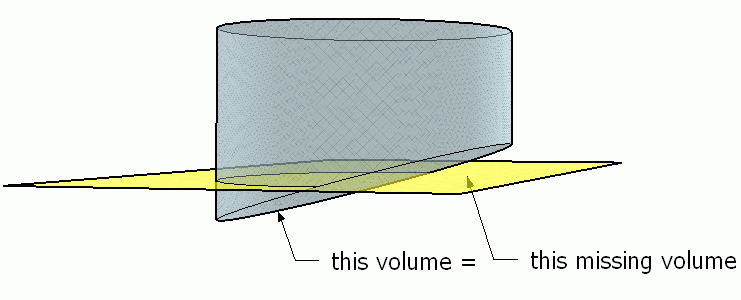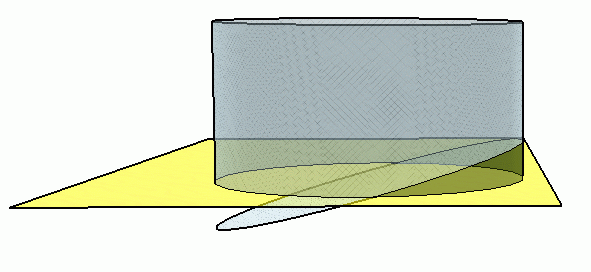SEARCH HOMEMath Central Quandaries & QueriesQuestion from ken: How much dirt do I need to fill a ground hole 20 ft in dia. with a sloping floor that has a ht. of 5" on one side and 12" on the other side?Hi Ken.

My diagrams are certainly not to scale. Let's mentally insert a horizontal plane to slice through the middle of the slope:By symmetry, it must be that the volume below the plane must equal the volume missing above the plane.So we can mentally slide it from below to above and we have a normal cylinder:Hence the space you want to fill is just the volume of a cylinder with diameter 20 ft and height equal to the midpoint of 5" and 12".

This makes the radius 120" and the height 8.5". The volume of any cylinder is πr2h, so the volume of this cylinder is π(120")2(8.5") = 384531 cubic inches.

In cubic yards, we have to multiply by (1 yd/36")3.

That's 384531×(1/36)3 = 8¼ cubic yards of dirt.

Regards,
Stephen La Rocque.Math Central is supported by the University of Regina and The Pacific Institute for the Mathematical Sciences.